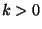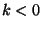IdentifiantMot de passe
Mot de passe oublié ?
19.1 Matlab(tm) compatible functions

# 19.1 Matlab(tm) compatible functions

numarray.mlab provides a set of Matlab(tm) compatible functions.

This will hopefully become a complete set of the basic functions available in Matlab. The syntax is kept as close to the Matlab syntax as possible. One fundamental change is that the first index in Matlab varies the fastest (as in FORTRAN). That means that it will usually perform reductions over columns, whereas with this object the most natural reductions are over rows. It's perfectly possible to make this work the way it does in Matlab if that's desired.

 mean( m, axis=0)
returns the mean along the axis'th dimension of m. Note: if m is an integer array, the result will be floating point. This was changed in release 10.1; previously, a meaningless integer divide was used.
 median( m)
returns a mean of m along the first dimension of m.
 min( m, axis=0)
returns the minimum along the axis'th dimension of m.
 msort( m)
returns a sort along the first dimension of m as in MATLAB.
 prod( m, axis=0)
returns the product of the elements along the axis'th dimension of m.
 ptp( m, axis = 0)
returns the maximum - minimum along the axis'th dimension of m.
 rand( d1, ..., dn)
returns a matrix of the given dimensions which is initialized to random numbers from a uniform distribution in the range [0,1).
 rot90( m,k=1)
returns the matrix found by rotating m by k*90 degrees in the counterclockwise direction.
 sinc( x)
returns sin(pi*x)
 squeeze( a)
removes any ones from the shape of a
 std( m, axis = 0)
returns the unbiased estimate of the population standard deviation from a sample along the axis'th dimension of m. (That is, the denominator for the calculation is n-1, not n.)
 sum( m, axis=0)
returns the sum of the elements along the axis'th dimension of m.
 svd( m)
return the singular value decomposition of m [u,x,v]
 trapz( y,x=None)
integrates y = f(x) using the trapezoidal rule
 tri( N, M=N, k=0, typecode=None)
returns a N-by-M matrix where all the diagonals starting from lower left corner up to the k-th are all ones.
 tril( m,k=0)
returns the elements on and below the k-th diagonal of m. k=0 is the main diagonal,is above andis below the main diagonal.

 triu( m,k=0)
returns the elements on and above the k-th diagonal of m. k=0 is the main diagonal,is above andis below the main diagonal.

Send comments to the NumArray community.